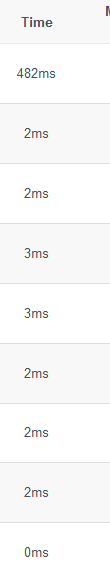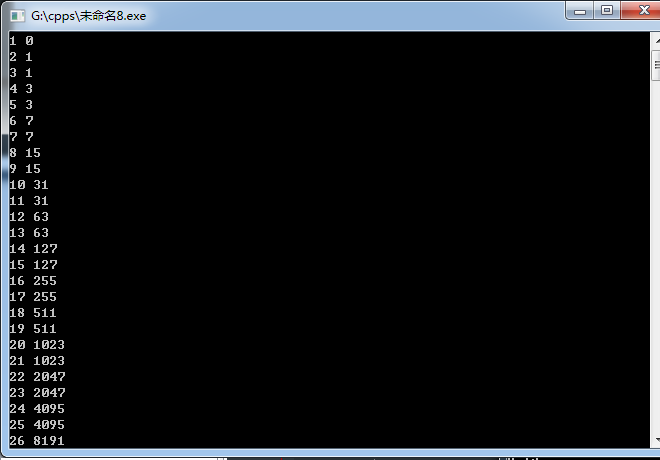Sorry, your browser cannot access this site

# 一堆递推题

——来自义冢OJ和义冢OJ的contests

## P1367【训练题】爬楼梯

### 輸入範例 1

5 2
2 4


### 輸出範例 1

2


### 提示

1 <= N <= 1000 0 <= k < N

### 思路

#include<cstdio>
#include<iostream>
using namespace std;

const int MAXN=1005;

int f[MAXN];
bool h[MAXN];

int main(){
int n,k;
cin>>n>>k;
for(int i=1;i<=k;++i){
int t;
cin>>t;
h[t]=1;
}
f=1;
//	f=1;
for(int i=1;i<=n;++i){
if(h[i]){
f[i]=0;
continue;
}
else if(!f[i]){
if(i-1>=0)f[i]+=f[i-1];
if(i-2>=0)f[i]+=f[i-2];
if(i-3>=0)f[i]+=f[i-3];
f[i]%=1234567;
}
}
cout<<f[n]<<endl;
return 0;
}


## 铺瓷砖

### 輸入範例 1

2


### 輸出範例 1

3


### 思路

f=f=1;

#include<cstdio>
#include<iostream>
using namespace std;

int f;

int main(){
int n;
cin>>n;
f=f=1;
//f=3;
for(int i=2;i<=n;++i){
f[i]=f[i-1]+f[i-2]*2;
f[i]%=12345;
}
cout<<f[n]<<endl;
return 0;
}


## 城市路径

### 輸入範例 1

4
0 0
8 3
11 -1
10 0


### 輸出範例 1

14


### 提示

【样例说明】跳过 2 号城市。【数据规模】对于 40% 的数据满足：n≤1000。对于 100% 的数据满足：3≤n≤100000，-1000≤x i ，y i ≤1000。

### 思路

? ——鲁迅

? ——hqxperisino大佬

#include<cstdio>
#include<cmath>
#include<iostream>
using namespace std;

const int MAXN=1e5+5;

struct zb{
int x,y;
} city[MAXN];
int n;

int main(){
scanf("%d",&n);
for(int i=1;i<=n;++i){
scanf("%d%d",&city[i].x,&city[i].y);
}
int maxs=0,maxi=0;
int he=0;

for(int i=2;i<n;++i){
int tmp=
abs(city[i+1].x-city[i].x)+abs(city[i+1].y-city[i].y)
+abs(city[i-1].x-city[i].x)+abs(city[i-1].y-city[i].y);
tmp-=abs(city[i+1].x-city[i-1].x)+abs(city[i+1].y-city[i-1].y);
if(tmp>maxs){
maxi=i;
maxs=tmp;
}
he+=abs(city[i-1].x-city[i].x)+abs(city[i-1].y-city[i].y);
}
he+=abs(city[n-1].x-city[n].x)+abs(city[n-1].y-city[n].y);
he-=maxs;
printf("%d\n",he);
return 0;
}


## 彩带

### 描述

(1) 相同颜色的彩带不能放在相邻的位置；

(2) 一条蓝色的彩带必须放在一条白色的彩带和一条红色的彩带中间。### 輸入範例 1

3


### 輸出範例 1

4


### 思路

f[i]为彩带数目为i时的方案数

• (i-1)条为红色或白色：因为最后一条不能是蓝色，所以有一种情况

• (i-1)条为蓝色：第i条与第(i-2)条颜色相反，有一种情况

• 大佬之前把第二个(i-1)写成了(i-2)我差点没看懂

#include<cstdio>
#include<iostream>
using namespace std;

const int MAXN=50;

int f[MAXN];
int n;

int main(){
f=2;
f=2;
cin>>n;
for(int i=3;i<=n;++i){
f[i]=f[i-1]+f[i-2];
}
cout<<f[n]<<endl;
return 0;
}


## 斐波那契前N项和

### 輸入範例 1

5 1000


### 輸出範例 1

12


### 提示

1<=n<=2*10^91<=m<=1000000010

### 思路

? 内容：f(i-1),f(i-2),g(i-1)

? 其中f(i)表示斐波那契数列的第i项，g(i)表示前i项的和

? 内容：

		1 1 1
1 0 1
0 0 1


$f(n)=A\cdot B^{(n-2)}$

#include<cstdio>
#include<cstring>
#include<iostream>
#define LL long long
using namespace std;

const LL MAXN=5;

LL n,m;

struct juzhen{
private:
LL v[MAXN][MAXN];
LL h,l;

void prLL(void){
for(LL i=1;i<=h;++i){
for(LL j=1;j<=l;++j){
cout<<v[i][j]<<' ';
}
cout<<'\n';
}
}

public:
juzhen(LL he,LL le){
memset(v,0,sizeof(v));
h=he,l=le;
}

friend juzhen operator *(juzhen a,juzhen b){
juzhen c(a.h,b.l);
for(LL i=1;i<=a.h;++i){
for(LL j=1;j<=b.l;++j){
for(LL k=1;k<=a.l;++k){
c.v[i][j]+=(a.v[i][k]*b.v[k][j]%m);
c.v[i][j]%=m;
}
}
}
return c;
}

friend juzhen operator ^(juzhen a,LL b){

juzhen ret(a.h,a.l),sum=a;
for(LL i=1;i<=a.h;++i){
ret.v[i][i]=1;
}
while(b){
if(b&1){
ret=ret*sum;
}
sum=sum*sum;
b>>=1;
}
//			ret.prLL();
return ret;
}

void writeV(LL x,LL y,LL val) {
v[x][y]=val;
}

LL callV(LL x,LL y){
return v[x][y];
}
};

juzhen a(1,3),b(3,3);
int main(void){
cin>>n>>m;
a.writeV(1,1,1);
a.writeV(1,2,1);
a.writeV(1,3,2);

b.writeV(1,1,1);
b.writeV(1,2,1);
b.writeV(1,3,1);
b.writeV(2,1,1);
b.writeV(2,2,0);
b.writeV(2,3,1);
b.writeV(3,1,0);
b.writeV(3,2,0);
b.writeV(3,3,1);

juzhen c=a*(b^(n-2));
cout<<c.callV(1,3)<<endl;

return 0;
}


## 偶数个3

### 輸入範例 1

2


### 輸出範例 1

73


### 思路

? 用f[i]表示i位数中有奇数个3的数量

f[i]=f[i-1]*9+f[i-1];
f[i]=f[i-1]*9+f[i-1];


## 回文拆分

### 描述

K=4时，有如下的拆分：

4=1+1+1+1 （回文数列1）

=1+1+2

=1+2+1 （回文数列2）

=2+1+1

=2+2 （回文数列3）

=1+3

=3+1

### 輸入範例 1

4


### 輸出範例 1

3


### 思路

? ——鲁迅

#include<cstdio>
#include<iostream>
using namespace std;

int f;

void dfs(int step,int tot);
int k,cnt;

int main(){
cin>>k;
dfs(1,0);
cout<<cnt<<endl;
return 0;
}

void dfs(int step,int tot){
if(tot>k)return;
if(tot==k){
bool flag=1;
for(int i=1;i<=(step-1)/2;++i){
if(f[i]!=f[step-i]){
flag=0;
break;
}
}
if(flag){
++cnt;
//			for(int i=1;i<step;++i){
//				cout<<f[i]<<' ';
//			}
//			cout<<'\n';
}
}
for(int i=1;i<=k-tot&&i<k;++i){
f[step]=i;
dfs(step+1,tot+i);
}
}for(int i=1;i<=26;++i){
cnt=0;
memset(f,0,sizeof(f));
k=i;
dfs(1,0);
cout<<i<<' '<<cnt<<endl;
}#include<cstdio>
#include<iostream>
using namespace std;

int qkpow(int x,int y);

int main(void){
int k;
cin>>k;
cout<<qkpow(2,k/2)-1<<endl;
return 0;
}

int qkpow(int x,int y){
if(y==0){
return 1;
}
int ret=1,sum=x;
while(y){
if(y&1){
ret*=sum;
}
sum*=sum;
y>>=1;
}
return ret;
}


~~